Next:9.7 Order StatisticsUp:9.6 Quick SortPrevious:9.6.2 Algorithm for Partitioning

## 9.6.3 Quicksort: Average Case Analysis

Assume that all initial orderings of the keys are equally likely;
• Assume that the keys are distinct

•

Note that the presence of equal keys will make the sorting easier, not harder.

• Also assume that when we call quicksort (i, j), all orders for A[i]SUP> ... A[j] are equally likely.

•

Let T(n) = average time taken by quicksort to sort n elements

• T(1) = C1 where C1 is some constant.
• Recall that the pivot is the larger of the first two elements.
• When n > 1, quicksort splits the subarray, taking C2ntime, where C2 is another constant.
Also, since the pivot is the larger of the first two elements, left groups tend to be larger than the right groups.

The left group can have i elements where i = 1, 2,..., n - 1, since the left group has at least one element and the right group also has at least one element.

Let us fix i and try to compute the probability:

P{ left group has i elements}

Now, left group has i elements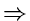pivot must be the (i + 1)stelement among the n elements

If the pivot is in position 1, then the element in position 2 is one of the i smaller elements and vice-versa.

P{ Position 1 contains one of the i smaller elements }

=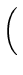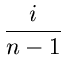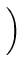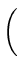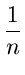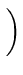Similarly,

P{ Position 2 contains one of the i smaller elements}

=Therefore,

P{ left group has i elements}

=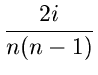T(nC2n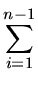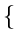T(i) + T(n - i)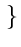Usingf (i) =f (n - i),
we get
T(nC2n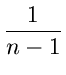T(i) + T(n - i)The above expression is in the form it would have been if we had picked a truly random pivot at each step.

The above simplifies to:
T(nC2n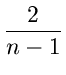T(i)
The above is the recurrence that one would get if all sizes between 1 and n - 1for the left group were equally likely. Thus picking the larger of the two elements doesn't really affect the size distribution.

We shall guess the solution
T(nCnlog n
for some constant C and prove its correctness using induction.

Basis:

n = 2Cnlog n = 2C

which is correct.

Induction Step:

AssumeT(i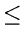Cilogi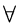i < n.

 T(n)C2n +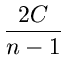ilog iC2n +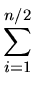ilogi +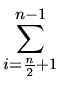ilog iC2n +ilog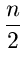+ilog nC2n + Cnlogn -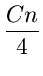-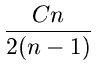,simplification

PickingC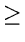4C2, we have,

C2n0
Thus T(n) is O(nlog n)Next:9.7 Order StatisticsUp:9.6 Quick SortPrevious:9.6.2 Algorithm for Partitioning
eEL,CSA_Dept,IISc,Bangalore